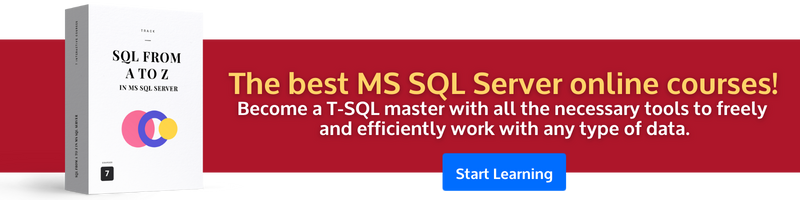# How to Convert an Integer to a Decimal in SQL Server

• CAST()
• CONVERT()

#### Problem:

You’d like to convert an integer value to a `DECIMAL` data type in SQL Server.

Let’s convert an integer to the `DECIMAL` data type.

#### Solution 1:

We’ll use the `CAST()` function. Here’s the query you’d write:```SELECT
CAST(12 AS DECIMAL(7,2)) AS decimal_value;
```

Here is the result:

decimal_value
12.00

#### Discussion:

Use the `CAST()` function to convert an integer to a `DECIMAL` data type. This function takes an expression or a column name as the argument, followed by the keyword AS and the new data type. In our example, we converted an integer (`12`) to a decimal value (`12.00`). The displayed value has two decimal points because `DECIMAL` in `CAST()` has two decimal points.

SQL Server provides another option: `CONVERT()`. This is not a SQL Standard function like `CAST()`. The query below shows its use.#### Solution 2:

Here’s another way to convert an integer to a `DECIMAL` type:

```SELECT
CONVERT(DECIMAL(7,2), 12) AS decimal_value;
```

This query produces the same result as `CAST()`, but takes two mandatory arguments: the data type and an expression, value, or column name to convert. An optional third parameter specifies how the value should be formatted in its new type. Read more about value formatting in the official SQL Server documentation.

If you don’t need to return the value in a certain format, use `CAST()`.